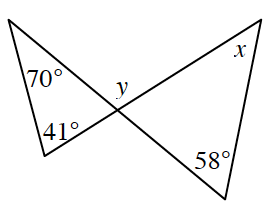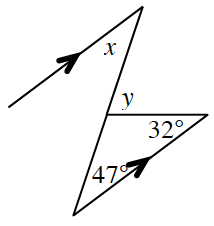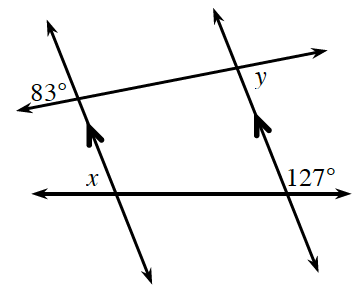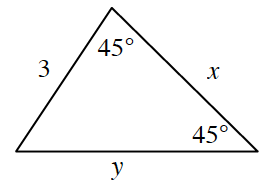### Home > CCG > Chapter 5 > Lesson 5.2.1 > Problem5-53

5-53.

Use the relationships found in each of the diagrams below to solve for $x$ and $y$. Assume the diagrams are not drawn to scale. State which geometric relationships you used.

1.Solve for the missing angle in the triangle with $70º$ and $41º$. Use the Triangle Angle Sum Theorem to solve for the missing angle.

$41º+70º+\text{missing angle}=180º$

$\text{missing angle}=69º$

$y$ and the $69º$ angle are supplementary angles, because they form a linear pair.

$69º+y=180º$

$y=111º$

The $69º$ angle and the angle across from it are vertical angles and vertical angles are congruent.

Using the Triangle Angle Sum Theorem and the triangle on the right, $69º+58º+x=180º$ so $x=53º$

1.$y=79º$, $x=47º$

1.Find the measures of any angles that may relate to the unknown angles by using the angles given.

1.This is a special right triangle. Try drawing it to scale and then solve for $x$ and $y$.